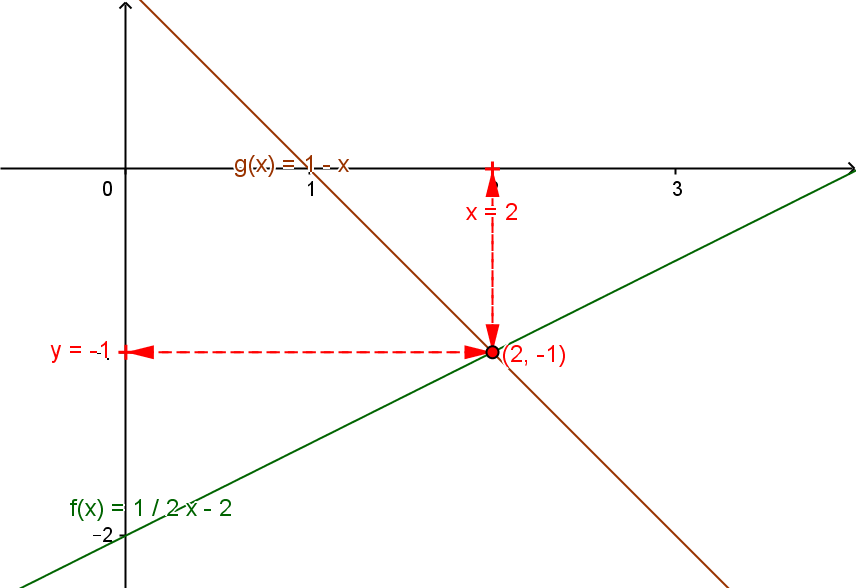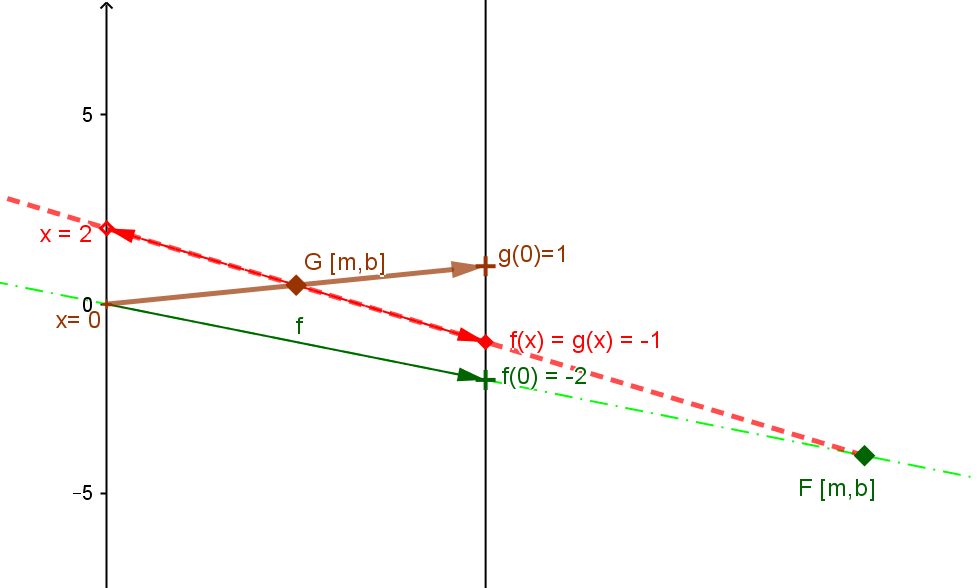Example LF.LEQ.6. Suppose $5x - 10y = 20$ (i) and $x+y=1$ (ii). Find $x$ and $y$.
Solution A. Using (ii) we see that $y=1-x$. Replace $y$ in the equation (i) by $1-x$ to give the equation $5*x -10*(1-x) = 15x- 10 = 20$ (iii).  This leads to the equation $15x = 30$ (iv) and thus the solution to the solution $x = 2$ and consequently $y=1-2=-1$..
Solution B. From (i) express $y$ as a function of $x$ giving $y = f(x) = \frac 5 {10} x - \frac {20} {10} = \frac 1 2 x - 2$ (v).  From (ii) express $y$ as  function of $x$ giving $y=g(x) =1-x$ .
Now find $x$ where $f(x) = g(x)$ or $1-x = \frac 1 2 x - 2$, which leads to $1= \frac 32 x -2$ and thus $x=2$ and $y=f(2)=g(2)= -1$.

In Solution B we find the linear functions being used to solve the problem by solving a related single linear equation based on the linear functions $f$ and $g$. This solution can be visualized both on the graph and the mapping diagram.

 For the graph of $f$ and $g$: Find the point at the intersection of the graphs of $f$ and $g$, $(2,-1)$, determine it's first coordinate, $2$, and that is the desired value for $x$, and its second coordinate, $-1$, is the desired value for $y$. For the mapping diagram of $f$ and $g$: Find the focus points of $f$, $F= [\frac 1 2, -2]$, and $g$, $G=[-1,1]$, on the mapping diagram. Draw the line through $F$ and $G$, to find the point of intersection of this line with the X axis, $x=2$, which is the desired value for $x$ and the Y axis, $y=-1$ for the desired value of $y$.